Trial ends in
JoVE Science Education
Mechanical Engineering

A subscription to JoVE is required to view this content.
You will only be able to see the first 20 seconds.

Buoyancy and Drag on Immersed Bodies
Hydraulic Jumps
Science Education (Mechanical Engineering)
Piping Networks and Pressure Losses

Buoyancy and Drag on Immersed Bodies

Overview

Source: Alexander S Rattner and Sanjay Adhikari; Department of Mechanical and Nuclear Engineering, The Pennsylvania State University, University Park, PA

Objects, vehicles, and organisms immersed in fluid mediums experience forces from the surrounding fluid in the form of buoyancy- a vertical upward force due to fluid weight, drag- a resistive force opposite the direction of motion, and lift- a force perpendicular to the direction of motion. Prediction and characterization of these forces is critical to engineering vehicles and understanding the motion of swimming and flying organisms.

In this experiment, the balance of buoyancy, weight, and drag forces on submerged bodies will be investigated by tracking the rise velocity of air bubbles and oil droplets in a glycerin medium. The resulting drag coefficients at terminal rise velocities will be compared with theoretical values.

Principles

When a body rises in a fluid medium, it experiences the external forces of gravity, buoyancy, and fluid drag. The force from gravity is weight (W), and acts downward with magnitude W = mg (m is the mass of the body, and g is the gravitational acceleration, 9.8 m s-2).

The buoyancy force (Fb) acts upward, opposing gravity. Pressure increases with depth in a fluid medium due to the greater weight of fluid above deeper points in the medium. Thus, the pressure force acting upward on the bottom of an immersed body is greater than the pressure force acting downward on the top of the body, resulting in the upward buoyancy force. The buoyancy force magnitude is Fb = ρfVg, where ρf is the density of the surrounding fluid medium and V is the volume of the immersed body. This is equal to the weight of fluid displaced by the submerged body.

When a body moves through a fluid medium, it experiences frictional resistance from the fluid, called drag. The drag force (FD) acts opposite to the direction of motion, and depends on the shape and size of the body, its velocity, and the fluid properties. In general, drag force can be modeled as: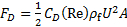(1)

Here, U is the velocity of the immersed body and A is the face area of the body (projected area in the direction of motion). CD is the drag coefficient, which depends on the shape of the body and its Reynolds number - a measure of the relative magnitude of inertial and viscous fluid forces on the body. Here,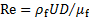, where D is a relevant length scale for the body (diameter for spheres and cylinders) and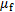is the fluid viscosity.

In this experiment, air bubbles and oil droplets will be injected into a high viscosity glycerin bath, and rise to the free surface. A free body diagram on a bubble/droplet (Fig. 1) rising at the terminal velocity (not accelerating) gives the vertical force balance: FB-W-FD = 0. Substituting earlier results, and assuming a spherical bubble (volume V = (1/6)πD3, face area A = (1/4)πD2) yields the following result (Eqn. 2). Here,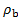is the density of the fluid inside the bubble/droplet.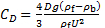(2)

In this experiment, the drag coefficient (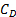) for spheres will be measured based on the rise velocity of different size bubbles and droplets. These data will be compared with the theoretical result of [1,2] for low Reynolds numbers (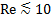).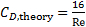(3)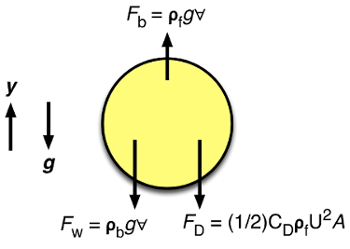Figure 1: Force balance on rising gas bubble or oil droplet

Procedure

1. Fabrication of gas injection test section (see schematic and photograph, Fig. 2)

1. Drill a hole in the bottom of a tall, flat walled plastic container. Install a through-wall bulkhead fitting through this hole. Install a reducing fitting to a ~3.2 mm tube compression connection in the bulkhead fitting outlet. This will be the bubble/droplet injection port.
2. Insert a short length (~1 cm) of 3.2 mm diameter soft rubber cord in the compression connection, and tighten the fitting nut. Using a sewing pin, puncture a thin hole through the rubber cord. This will be the valve for injecting bubbles/droplets into the fluid container.
3. Fill the container with glycerin to a level of ~25 cm. Pour the glycerin slowly as a film down the container sidewall to help reduce bubble entrainment in the container. Wait for ~2 hours to allow larger bubbles to rise out of the container.
4. Mount a video camera on a tripod facing the container, with the upper portion of the liquid in view. Mount a bright light on the other side of the container, facing the camera (backlighting). Insert a diffuser sheet between the light and container to ensure even illumination.

2. Performing experiments

1. Insert a ruler or flat object of known size into the glycerin container, above the injection port, facing the camera. Record a brief video of the object. This will serve a scale to to map from bubble size in px and rise velocity in px s-1 to m and m s-1, respectively.
2. Using a syringe with a thin needle (e.g., 20 gauge). Inject gas bubbles of varying sizes through the rubber valve into the liquid. Use the camera to record videos of the bubbles rising through the liquid.
3. Mix oil-based food coloring with soybean vegetable oil (or other low-viscosity vegetable oil). Using the syringe, inject colored oil droplets of varying sizes into the glycerin container. Record videos of the droplets rising.

3. Analysis

1. Using software such as VLC media player, export image snapshots from the video of the ruler (Step 2.1). In an image editing software measure the pixel distance across a known length of the device. The length scaling factor can then be determined as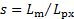, where Lm is the physical length of the object in meters and Lpx is the object length in pixels in the image.
2. For each bubble or droplet rise velocity video, extract image snapshots from when the bubbles/droplets enter and exit the camera view window. Measure the bubble/droplet (horizontal) diameters in an image editing software (Dpx). Measure the average rise velocities (Upx) as the difference in bubble/droplet nose positions divided by elapsed video times between initial and final image snapshots. Convert these pixel values to physical values as: D = sDpx and U = sUpx.
3. Evaluate bubble and droplet Reynolds numbers () and drag coefficients (Eqn. 2). Plot these values and compare with theoretical results from Eqn. 3. Fluid properties at room temperature (22°C) are:
• Glycerin: ρf = 1300 kg m-3, µf = 3.7 kg m-1 s-1
• Air: ρb = 1.19 kg m-3
• Soybean oil: ρb = 920 kg m-3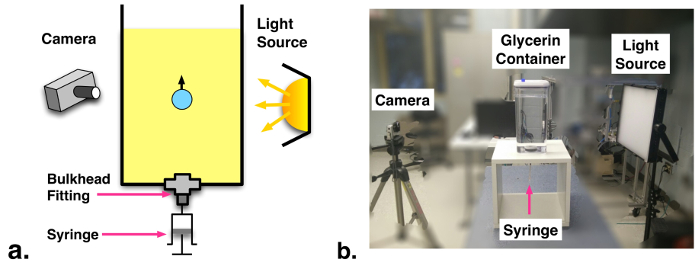Figure 2: (a) Schematic and (b) photograph of experimental facility.

Buoyancy and drag are two forces that commonly arise when considering the motion of an object through a fluid. The prediction and characterization of these forces is critical to solving many mechanical problems, such as engineering vehicles, or understanding the motion of swimming and flying organisms. As your intuition might suggest, the buoyant force acts vertically upward on the object in direct opposition to gravity. Likewise, the drag force tends to slow an object down relative to the surrounding fluid, acting in opposition to the relative motion of the object. In this video, these two forces will be examined in greater detail to show how they arise and how to determine their magnitude. Their effect on small bubbles and droplets rising in a fluid will then be illustrated by an experiment before finishing with a discussion of other applications.

To begin, let's have a closer look at buoyancy. When an object is fully immersed in a fluid, the magnitude of the buoyant force is simply the product of the surrounding fluid density, the volume of the object, and the acceleration due to gravity. This is equivalent to the weight of the fluid displaced by the object, as stated by Archimedes' Principle. Of course, the gravitational force, which is the average density of the object times it's volume and acceleration due to gravity, is still pulling downward in opposition to the buoyant force. So, if the average density of the object equals the density of the fluid, the sum of the buoyant and gravitational forces will equal zero, and the object will be neutrally buoyant. Likewise, if the object is more dense, it will sink, and if it is less dense, it will float. Once the object begins moving however, it will encounter another force, drag. Drag is due to frictional resistance caused by the motion of the object through the fluid, and acts against the direction of motion as indicated by the velocity vector "U". Calculating the magnitude of the drag force is more complicated, but in general, it can be modeled as 1/2 the product of the fluid density, the projected area of the body and the direction of motion, the drag coefficient, and the relative velocity squared. The drag coefficient captures the effect of the shape of the object and since it depends on the Reynolds Number, also takes into account the relative magnitude of inertial and viscous fluid forces on the body. The Reynolds Number is determined by multiplying the relative velocity and characteristic length scale of the object, by the ratio of the fluids density and viscosity, but in general, there is no simple equation for the drag coefficient, and it must be determined empirically or numerically. Now, consider all three of these forces acting on a spherical object in a dense fluid. The buoyant force will counter the force of gravity, and accelerate the object upwards. But as velocity increases, so will the drag. Eventually, the object will reach a constant velocity, called the Terminal Velocity, where all three forces are in balance. If the density of the fluid and the mass diameter and terminal velocity of this sphere are known, then the drag coefficient can be calculated. Now, let's test these principles by measuring the drag coefficient of small air bubbles in oil droplets rising in glycerin, and comparing the results to theory. For low Reynolds Number bubbles and droplets, the drag coefficient should be 16 divided by the Reynolds Number.

To perform these tests, you'll need a clear liquid tank with an injection port. Follow the instructions in the text to assemble the tank. When construction of the tank is complete, set it up so that the injection port is easily accessible, and the fill it with glycerin to a depth of approximately 25 cm by slowly pouring a film against the inside wall. This technique will help to reduce bubble entrainment in the container. Some gas will inevitably get entrained and will need time to rise out of the glycerin, so use this time to set up the camera and backlight. Affix the camera to a tripod, facing the container squarely and high enough that the upper portion of the liquid is in view. Opposite the camera, mount a bright light source, and if necessary, insert a diffuser sheet between the light and the container to achieve more even illumination. Now, carefully insert a ruler vertically into the glycerin above the injection port, with the markings facing the camera. Adjust the field of view to span a vertical height of approximately 150mm, and the focus the camera on the markings. Record a brief video of the ruler for calibration, and then carefully extract it from the tank. Do not adjust the position or field of view of the camera for the remainder of the experiment or the calibration will be invalid. Finally, prepare two syringes with thin needles. The first syringe will just contain air, but fill the second with a mixture of a low viscosity vegetable oil and an oil based food coloring. You are now ready to perform the experiment. Use the first syringe to inject an air bubble, and record it with the camera as it rises. Repeat this process 10 to 15 times, and with a variety of bubble sizes. Now, repeat the procedure with the colored oil and record 10 to 15 droplets of varying size.

Transfer all of the video files from the camera to a computer with software capable of exporting individual frames from the videos as images. Open the calibration video of the ruler first and export one frame. Use this image to determine the scaling factor in terms of meters per pixel. After you have the scaling factor, you can process the rest of the videos. Export one frame with the bubble or droplet near the bottom of the view and measure the horizontal diameter in pixels. Next, measure the vertical distance in pixels from the top of the image to the top edge of the bubble or droplet. Finally, record the timestamp for this frame. Now, export a second frame with the bubble or droplet near the top of the view, but still completely within the glycerin. Once again, measure the horizontal diameter, the vertical distance, and the timestamp. You now have two horizontal diameters and vertical positions corresponding to the two measurement times. Take the average of the diameter measurements, and then use the scaling factor to convert this value from pixels to meters. Now, take the difference in vertical height between the two frames. Use the scaling factor once again to convert this distance from pixels to meters. The time taken to rise this distance is found by taking the difference between timestamps for the two frames. Now that the changes in position and time are known, the terminal velocity is easily determined by taking the ratio of the two. Use these results to calculate the drag coefficient with the equation that was derived earlier. Look up published values for the fluid densities and the acceleration due to gravity. Recall that the theoretical treatment predicts a relationship between the drag coefficient and the Reynolds number. Calculate the Reynolds number using your measurements and the published values for the density and viscosity of glycerin. We will use this result soon to compare the measurements with theory, but for a meaningful comparison, the measurement uncertainty must also be known. Propagate your uncertainties as described in the text to determine the final uncertainty in the drag coefficient and the Reynolds number. Once you have finished analyzing all of the videos, take a look at the results.

First, compare the videos from air bubbles of different sizes. At these low velocity and length scales, strong surface tension forces result in nearly spherical bubbles, but the smaller bubbles rise at lower velocities due to relatively stronger drag forces. The largest bubbles approach a Reynolds number of two resulting in somewhat flattened tails in the wake region. Now, compare the videos of different sizes of oil droplets. As with the bubbles, the droplets remain nearly spherical, and the smaller droplets rise at lower velocities due to stronger drag forces. The largest oil drops only approach a Reynolds number of 0.2 however due to their greater weight, and they form slightly teardrop shapes, likely due to the high inertia of the oil circulating inside the droplets. Finally, plop the measured drag coefficient as a function of Reynolds number for the bubbles and droplets, and compare this to the theoretical prediction. Overall, qualitatively close agreement is observed with the theory with most measured drag coefficient values matching within experimental uncertainty.

Buoyancy and drag are forces that impact an enormous variety of industrial processes and mechanical systems. Boiling water reactors, BWRs, are a type of steam generator in nuclear power plants. In these reactors, vertical bundles of radioactive fuel rods heat upward flowing high-pressure water to produce steam. This video shows a scaled down experiment of liquid gas flow along transparent cylinders representing the fuel rods. Concepts such as buoyancy and drag must be considered to predict the behavior of two phase flow in these fuel assemblies and ensure safe operation. If gas bubbles are not removed quick enough by buoyancy and fluid flow, the fuel rods surfaces can dry out, leading to over-heating and failure. Vehicles such ass cars, planes, and boats experience significant drag forces. For example, at highway speeds a typical sedan may require horsepower or 30 kW, just to overcome aerodynamic resistance. Careful design on vehicle shape and intake exhaust pathways can control airflow around a vehicle and reduce drag. Thereby, increasing efficiency.

You've just watched Jove's Introduction to Buoyancy and Drag. You should now understand how and when these forces arise and how they can effect the motion of objects in a fluid. You have seen how to calculate these forces based on physical properties and a method for determining the drag coefficient of an object by measuring its terminal velocity. Thanks for watching.

Results

A series of rising air bubbles and oil droplets of varying diameters are presented in Fig. 3. The small bubbles and droplets rise at lower velocities due to relatively stronger drag forces. At these low velocity and length scales, strong surface tension forces result in nearly spherical bubbles and droplets. The largest bubbles approach Re ~ 2, resulting in somewhat flattened tails in the wake region. The largest oil droplets only approach Re ~ 0.2 due to their greater weights. The large droplets form slightly teardrop shapes, likely due to the high inertia (density) of the oil circulating inside the droplets. In contrast, the low density air in the gas bubbles has negligible inertia.

Measured drag coefficients (Eqn. 2) are compared with theoretical values for air bubbles and oil droplets (Eqn. 3) in Fig. 4. The most significant sources of uncertainty in this study stem from the glycerin viscosity value, which varies sharply with temperature, and the diameters of the smallest bubbles/droplets. Here, uncertainty propagation is performed assuming ± 0.2 kg m-1 s-1 for glycerin viscosity (corresponds to ~ ±1°C) and ±1.5 mm for bubble diameter (~3 px). Overall, qualitatively close agreement is observed with theory in Fig. 4, with most measured CD values matching theoretical results within experimental uncertainty.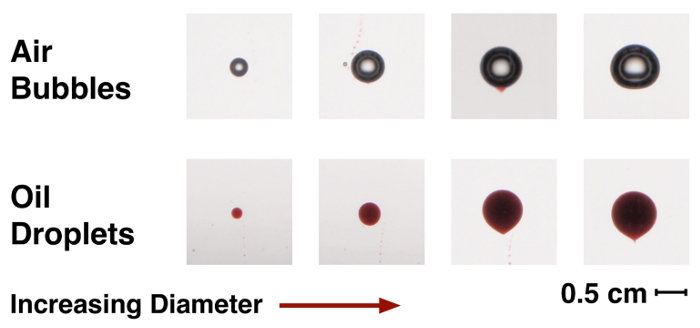Figure 3: Image series of rising gas bubbles and oil droplets of varying diameters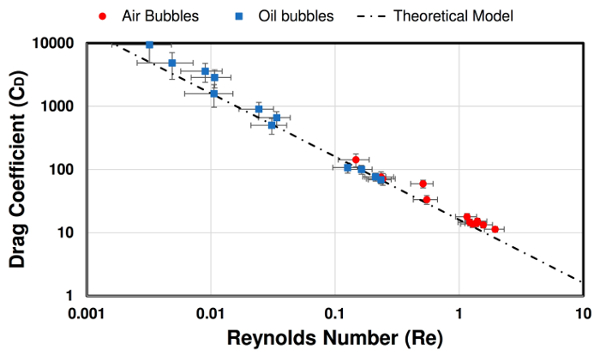Figure 4: Measured drag coefficients and Reynolds number for rising bubbles and droplets compared with theoretical model (Eqn. 3).

Applications and SummaryX